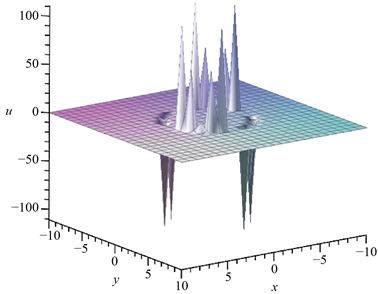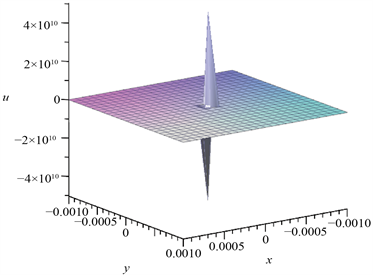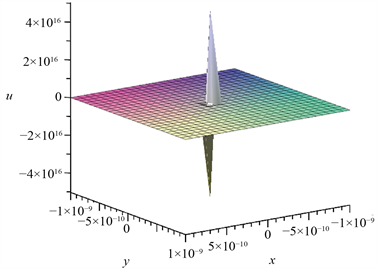﻿ (2 + 1)维变系数Broer-Kaup方程的精确解与局域激发

# (2 + 1)维变系数Broer-Kaup方程的精确解与局域激发Exact Solution and Local Excitation of (2 + 1)-Dimensional Variable Coefficient Broer-Kaup Equation

Abstract: The extended Riccati mapping method is used to analyze the 2 + 1 dimensional variable coefficient Broer-Kaup equation, and the exact solution containing any function is obtained. By selecting the arbitrary function, the relevant local excitation structure is obtained. This method is also widely used in solving other nonlinear partial differential equations.

1. 引言

${u}_{yt}-\alpha \left(t\right)\left[{u}_{xxy}-2{\left(u{u}_{x}\right)}_{y}-2{v}_{xx}\right]=0$

${v}_{t}+\alpha \left(t\right)\left[{v}_{xx}+2{\left(uv\right)}_{x}\right]=0$ (1.1)

2. 拓展的Riccati展开法解法概述

$P\left(u,{u}_{t},{u}_{x},{u}_{xx}{u}_{xt},\cdots \right)$ (2.1)

$u=\underset{i=-n}{\overset{n}{\sum }}{a}_{i}\left(x\right){\varphi }^{i}\left(q\right)$ (2.2)

${\varphi }^{\prime }=\sigma +{\varphi }^{2}$ (2.3)

(2.3)解的情况如下

$\begin{array}{l}\varphi =\sqrt{\sigma }\mathrm{tan}\left(\sqrt{\sigma }q\right)\text{\hspace{0.17em}}\text{\hspace{0.17em}}\text{\hspace{0.17em}}\text{\hspace{0.17em}}\text{\hspace{0.17em}}\sigma >0\\ \varphi =-\sqrt{\sigma }\mathrm{cot}\left(\sqrt{\sigma }q\right)\text{\hspace{0.17em}}\text{\hspace{0.17em}}\text{\hspace{0.17em}}\sigma >0\end{array}$

$\begin{array}{l}\varphi =-\sqrt{-\sigma }\mathrm{tanh}\left(\sqrt{-\sigma }q\right)\text{\hspace{0.17em}}\text{\hspace{0.17em}}\text{\hspace{0.17em}}\text{\hspace{0.17em}}\sigma <0\\ \varphi =-\sqrt{-\sigma }\mathrm{coth}\left(\sqrt{-\sigma }q\right)\text{\hspace{0.17em}}\text{\hspace{0.17em}}\text{\hspace{0.17em}}\text{\hspace{0.17em}}\sigma <0\end{array}$

$\varphi =-1/q\text{\hspace{0.17em}}\text{\hspace{0.17em}}\text{\hspace{0.17em}}\text{\hspace{0.17em}}\text{\hspace{0.17em}}\text{\hspace{0.17em}}\sigma =0$ (2.4)

3. 2 + 1维变系数BK方程的精确解

$v={u}_{y}$ (3.1)

${u}_{yt}+\alpha \left(t\right)\left[2{\left(u{u}_{x}\right)}_{y}+{u}_{xxy}\right]=0$ (3.2)

$u=f+g\varphi \left(q\right)+h{\varphi }^{-1}\left(q\right)$ (3.3)

$f=-\frac{\alpha \left(t\right){q}_{xx}+{q}_{t}}{2{q}_{x}\alpha \left(t\right)}$$g=-{q}_{x}$$h={q}_{x}\sigma$ (3.4)

$q\left(x,y,t\right)=\chi \left(x,t\right)+\phi \left(y\right)$ (3.5)

${u}_{1}=-\frac{\alpha \left(t\right){\chi }_{xx}+{\chi }_{t}}{2{\chi }_{x}\alpha \left(t\right)}+{\chi }_{x}\cdot \sqrt{-\sigma }\mathrm{tanh}\left(\sqrt{-\sigma }\left(\chi +\phi \right)\right)-{\chi }_{x}\sigma \cdot {\left(\sqrt{-\sigma }\mathrm{tanh}\left(\sqrt{-\sigma }\left(\chi +\phi \right)\right)\right)}^{-1}$ (3.6)

${v}_{1}=-{\chi }_{x}\sigma {\phi }^{\prime }\left({\text{sech}}^{2}\left(\sqrt{-\sigma }\left(\chi +\phi \right)\right)-\frac{{\text{sech}}^{2}\left(\sqrt{-\sigma }\left(\chi +\phi \right)\right)}{{\mathrm{tanh}}^{2}\left(\sqrt{-\sigma }\left(\chi +\phi \right)\right)}\right)$ (3.7)

${u}_{2}=-\frac{\alpha \left(t\right){\chi }_{xx}+{\chi }_{t}}{2{\chi }_{x}\alpha \left(t\right)}+{\chi }_{x}\cdot \sqrt{-\sigma }\cdot \mathrm{coth}\left(\sqrt{-\sigma }\left(\chi +\phi \right)\right)-{\chi }_{x}\sigma \cdot {\left(\sqrt{-\sigma }\cdot \mathrm{coth}\left(\sqrt{-\sigma }\left(\chi +\phi \right)\right)\right)}^{-1}$ (3.8)

${v}_{2}={\chi }_{x}\sigma {\phi }^{\prime }\left({\text{csch}}^{2}\left(\sqrt{-\sigma }\left(\chi +\phi \right)\right)-\frac{{\text{csch}}^{2}\left(\sqrt{-\sigma }\left(\chi +\phi \right)\right)}{{\mathrm{coth}}^{2}\left(\sqrt{-\sigma }\left(\chi +\phi \right)\right)}\right)$ (3.9)

${u}_{3}=-\frac{\alpha \left(t\right){\chi }_{xx}+{\chi }_{t}}{2{\chi }_{x}\alpha \left(t\right)}-{\chi }_{x}\cdot \sqrt{\sigma }\mathrm{tan}\left(\sqrt{\sigma }\left(\chi +\phi \right)\right)+{\chi }_{x}\sigma \cdot {\left(\sqrt{\sigma }\mathrm{tan}\left(\sqrt{\sigma }\left(\chi +\phi \right)\right)\right)}^{-1}$ (3.10)

${v}_{3}=-{\chi }_{x}\sigma {\phi }^{\prime }\left({\mathrm{sec}}^{2}\left(\sqrt{\sigma }\left(\chi +\phi \right)\right)+\frac{{\mathrm{sec}}^{2}\left(\sqrt{\sigma }\left(\chi +\phi \right)\right)}{{\mathrm{tan}}^{2}\left(\sqrt{\sigma }\left(\chi +\phi \right)\right)}\right)$ (3.11)

${u}_{4}=-\frac{\alpha \left(t\right){\chi }_{xx}+{\chi }_{t}}{2{\chi }_{x}\alpha \left(t\right)}+{\chi }_{x}\cdot \sqrt{\sigma }\mathrm{cot}\left(\sqrt{\sigma }\left(\chi +\phi \right)\right)-{\chi }_{x}\sigma \cdot {\left(\sqrt{\sigma }\mathrm{cot}\left(\sqrt{\sigma }\left(\chi +\phi \right)\right)\right)}^{-1}$ (3.12)

${v}_{4}=-{\chi }_{x}\sigma {\phi }^{\prime }\left({\mathrm{csc}}^{2}\left(\sqrt{\sigma }\left(\chi +\phi \right)\right)+\frac{{\mathrm{csc}}^{2}\left(\sqrt{\sigma }\left(\chi +\phi \right)\right)}{{\mathrm{cot}}^{2}\left(\sqrt{\sigma }\left(\chi +\phi \right)\right)}\right)$ (3.13)

${u}_{5}=-\frac{\alpha \left(t\right){\chi }_{xx}+{\chi }_{t}}{2{\chi }_{x}\alpha \left(t\right)}+{\chi }_{x}\cdot \frac{1}{\chi +\phi }-{\chi }_{x}\sigma \cdot \left(\chi +\phi \right)$ (3.14)

${v}_{5}=-{\chi }_{x}\cdot \frac{{\phi }^{\prime }}{{\left(\chi +\phi \right)}^{2}}-{\chi }_{x}\sigma \cdot {\phi }^{\prime }$ (3.15)

4. 局域激发与分形结构

$\chi \left(x,t\right)=-{x}^{2}+{t}^{2}$$\phi \left(y\right)=-{y}^{2}$ (3.16)Figure 1. The ring soliton structure is obtained when $t=4$, $\sigma =1$Figure 2. Take $t=0$, $\sigma =1$ Take to get the light and dark domionFigure 3. Figure 2 takes $x,y\in \left[-1×{10}^{-6},1×{10}^{-6}\right]$Figure 4. Figure 2 takes $x,y\in \left[-1×{10}^{-9},1×{10}^{-9}\right]$

5. 结论

 李志斌. 非线性数学物理方程的行波解[M]. 北京: 科学出版社, 2007.

 楼森岳, 唐晓艳. 非线性数学物理方法[M]. 北京: 科学出版社, 2006.

 刘丽环, 常晶, 冯雪. 求非线性发展方程行波解的(G′/G)展开法[J]. 吉林大学学报(理学版), 2013, 51(200): 31-34.

 张楠鸽, 马松华. 变系数(2 + 1)维Broer-Kaup方程的复合波激发及分形结构[J]. 丽水学院学报, 2014, 36(5): 21-28.

 洪宝剑, 卢殿臣. 变系数(2 + 1)维Broer-Kaup方程新的类孤子解[J]. 原子与分子物理学报, 2008, 25(1): 136-140.

 郑春龙. (2 + 1)维非线性系统的局域激发模式及其分形和混沌行为研究[D]: [博士学位论文]. 上海: 上海大学, 2005.

 黄文华, 卢颖. (2 + 1)维高阶Broer-Kaup方程的多种局域相干结构(英文) [J]. 湖州师范学院学报, 2018, 40(276): 6-11+34.

 白玉梅, 套格图桑. 变系数(2 + 1)维Broer-Kaup方程的无穷序列新精确解[J]. 内蒙古师范大学学报(自然科学汉文版), 2015, 44(176): 28-33.

 马志民, 孙峪怀. (2 + 1)维变系数Broer-Kaup系统的精确解[J]. 广西科学院学报, 2013, 29(100): 14-16.

 Wang, L.H. and He, J.S. (2012) Integrability and Solutions of the (2 + 1)-Dimensional Broer-Kaup Equation with Variable Coefficients. Communications in Theoretical Physics, 58, 75-80.
https://doi.org/10.1088/0253-6102/58/3/11

 包来友, 包志华. 变系数(2 + 1)维Broer-Kaup方程的分离变量解[J]. 呼伦贝尔学院学报, 2011, 19(90): 83-85.

 刘丽红. (2 + 1)维耗散长水波方程和Broer-Kaup方程的显示解[J]. 吉林师范大学学报(自然科学版), 2011, 32(115): 65-68+71.

 许韬. 符号计算与若干非线性发展方程的解析研究[D]: [博士学位论文]. 北京: 北京邮电大学, 2011.

 刘萍. Broer-Kaup系统的达布变换及其偶孤子解(英文) [J]. 西南大学学报(自然科学版), 2009, 31(175): 69-74.

Top